# RD Sharma Solutions for Class 11 Chapter 5 - Trigonometric Functions Exercise 5.3

In Exercise 5.3, we shall discuss problems based on signs of trigonometric functions and variations in values of trigonometric functions in different quadrants. Students can refer to RD Sharma Class 11 Solutionswhich is designed by our expert tutors for the purpose of strengthening their concepts and problem-solving abilities. Solutions here are developed with utmost care to help students analyze the problems competently and hence, score good marks in their exams. Students can download the pdfs of RD Sharma Class 11 from the links provided below and start practising offline.

## Download the Pdf of RD Sharma Solutions for Class 11 Maths Exercise 5.3 Chapter 5 – Trigonometric Functions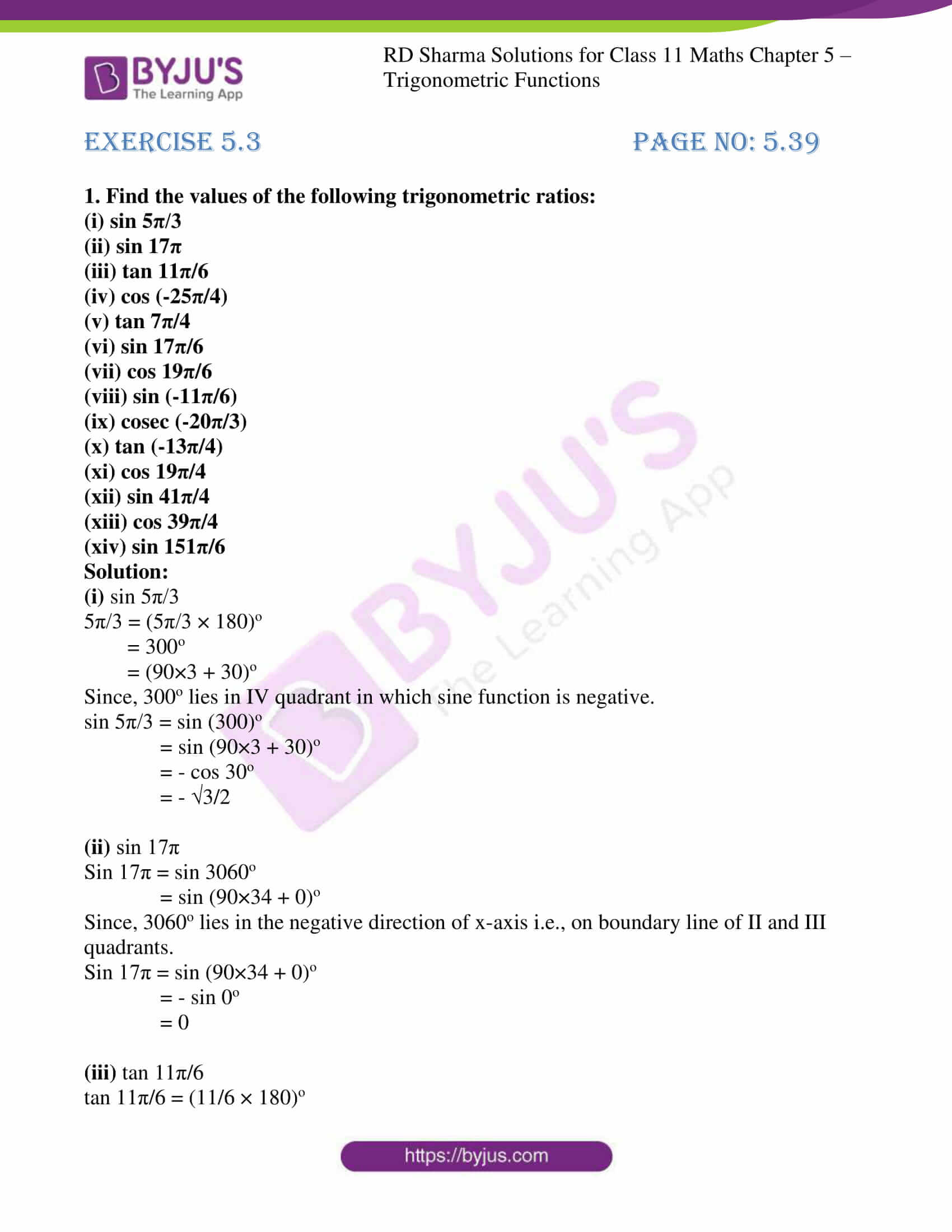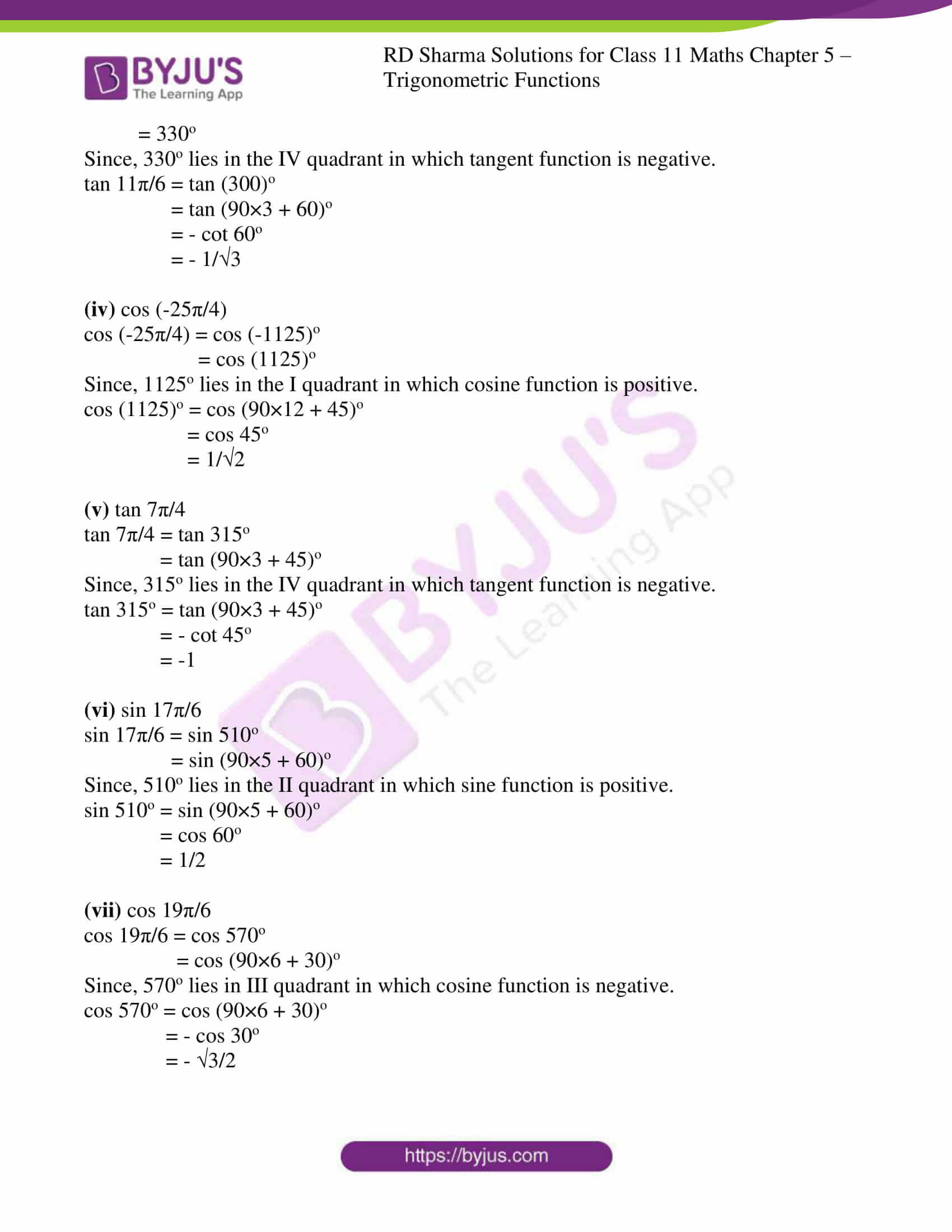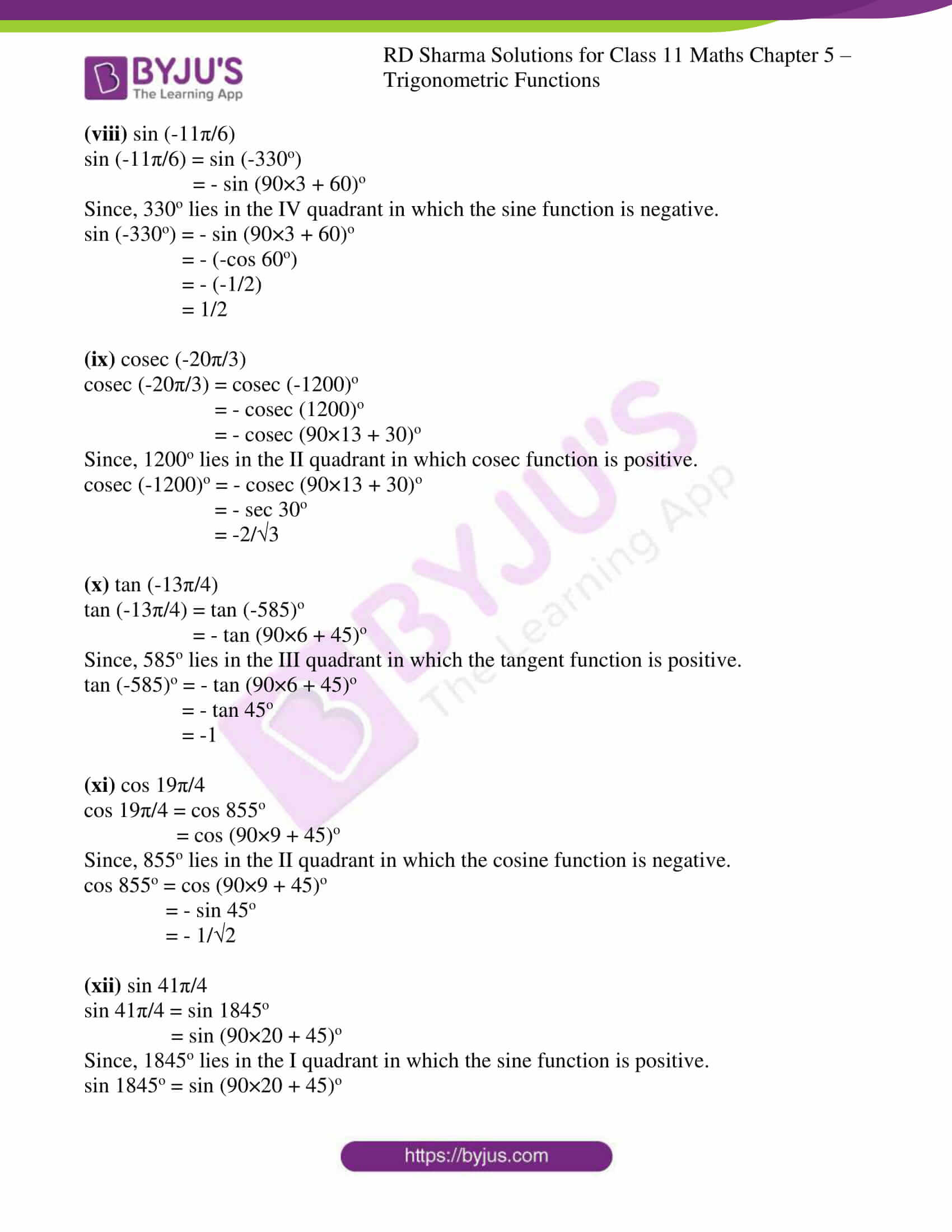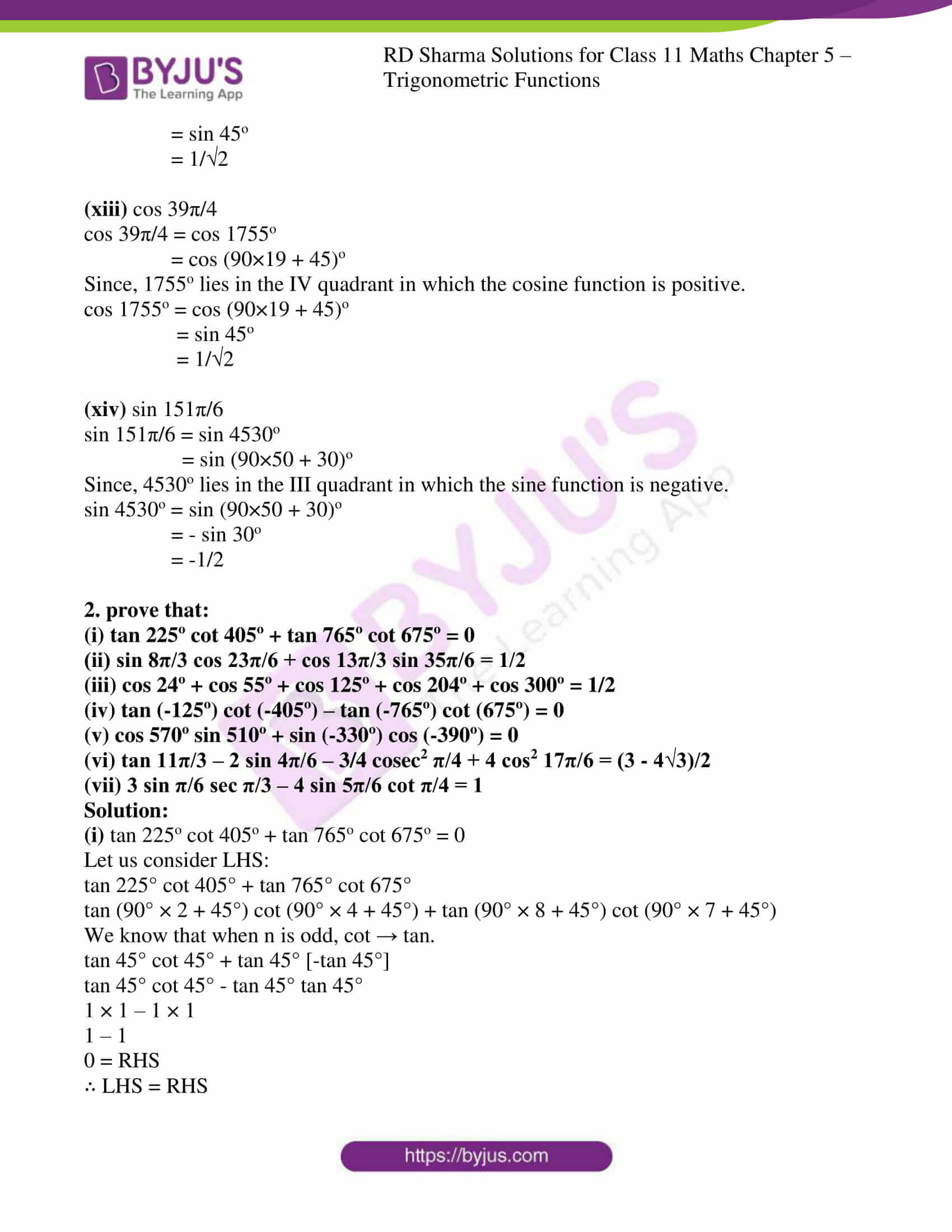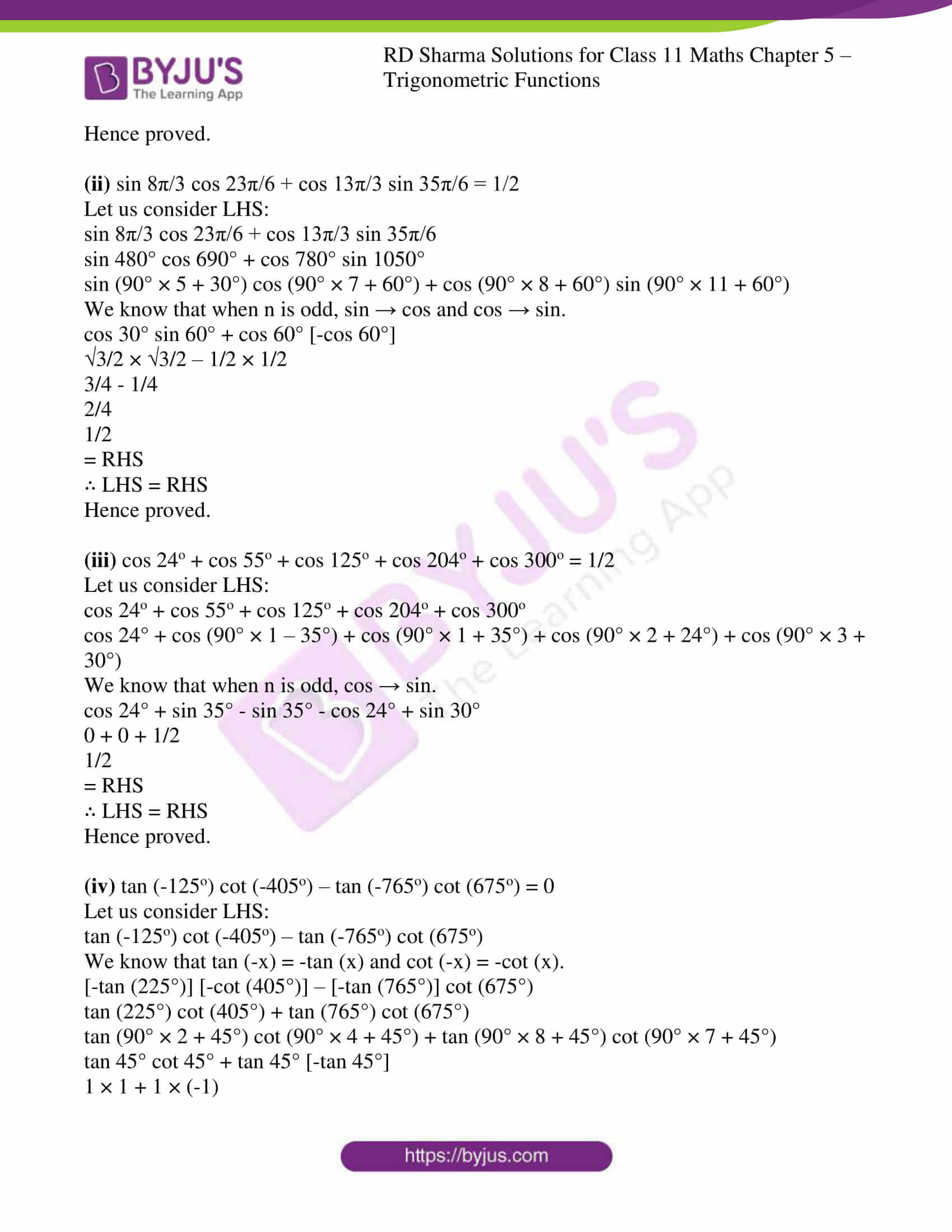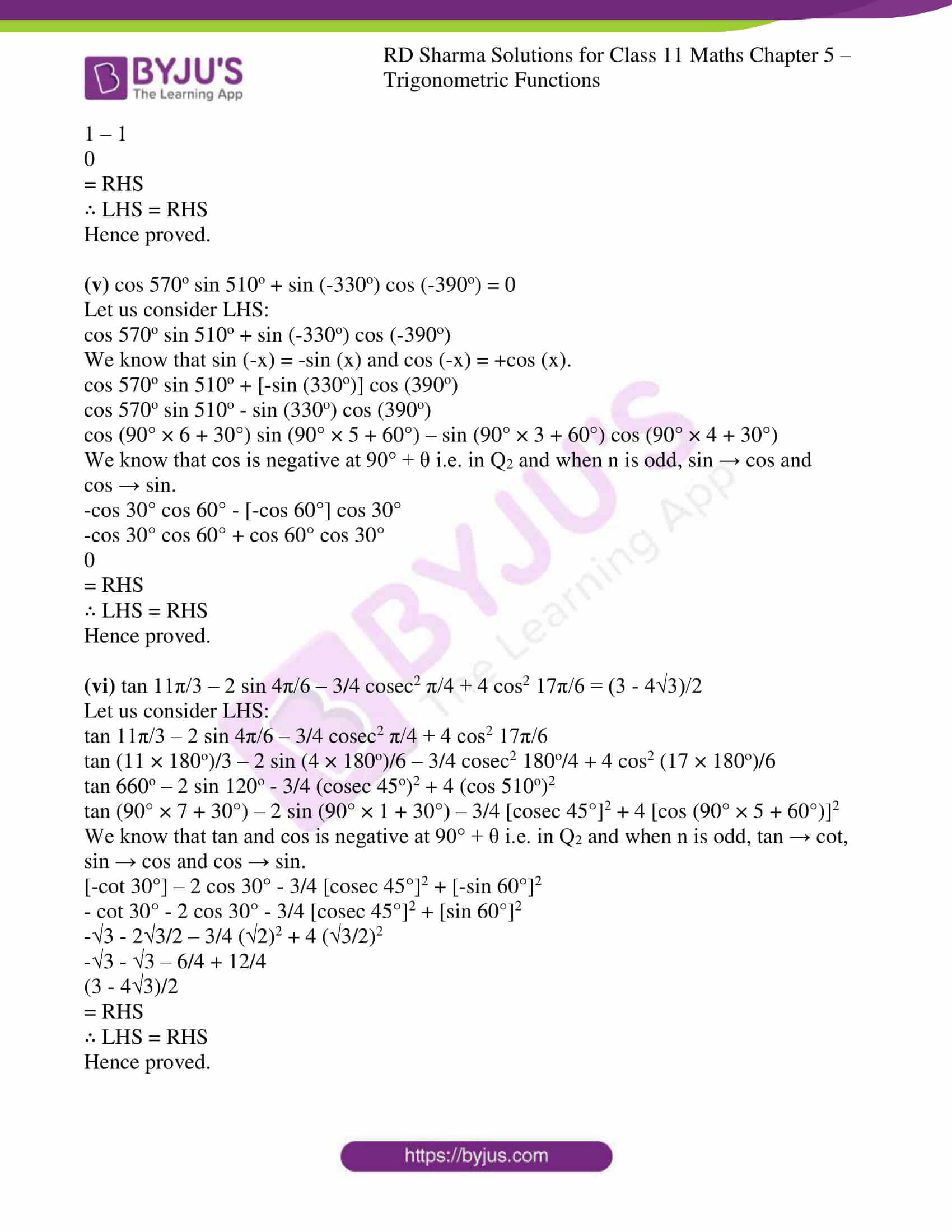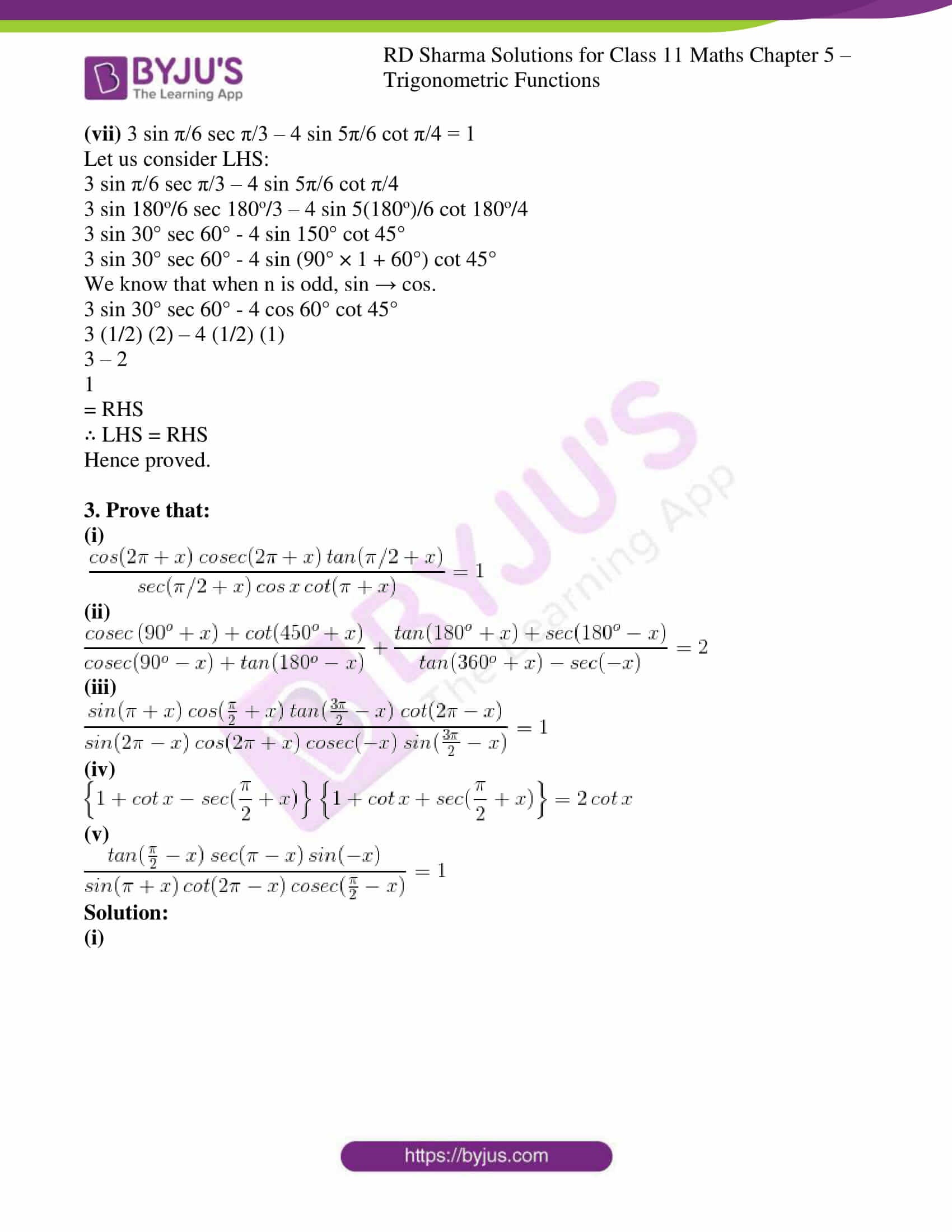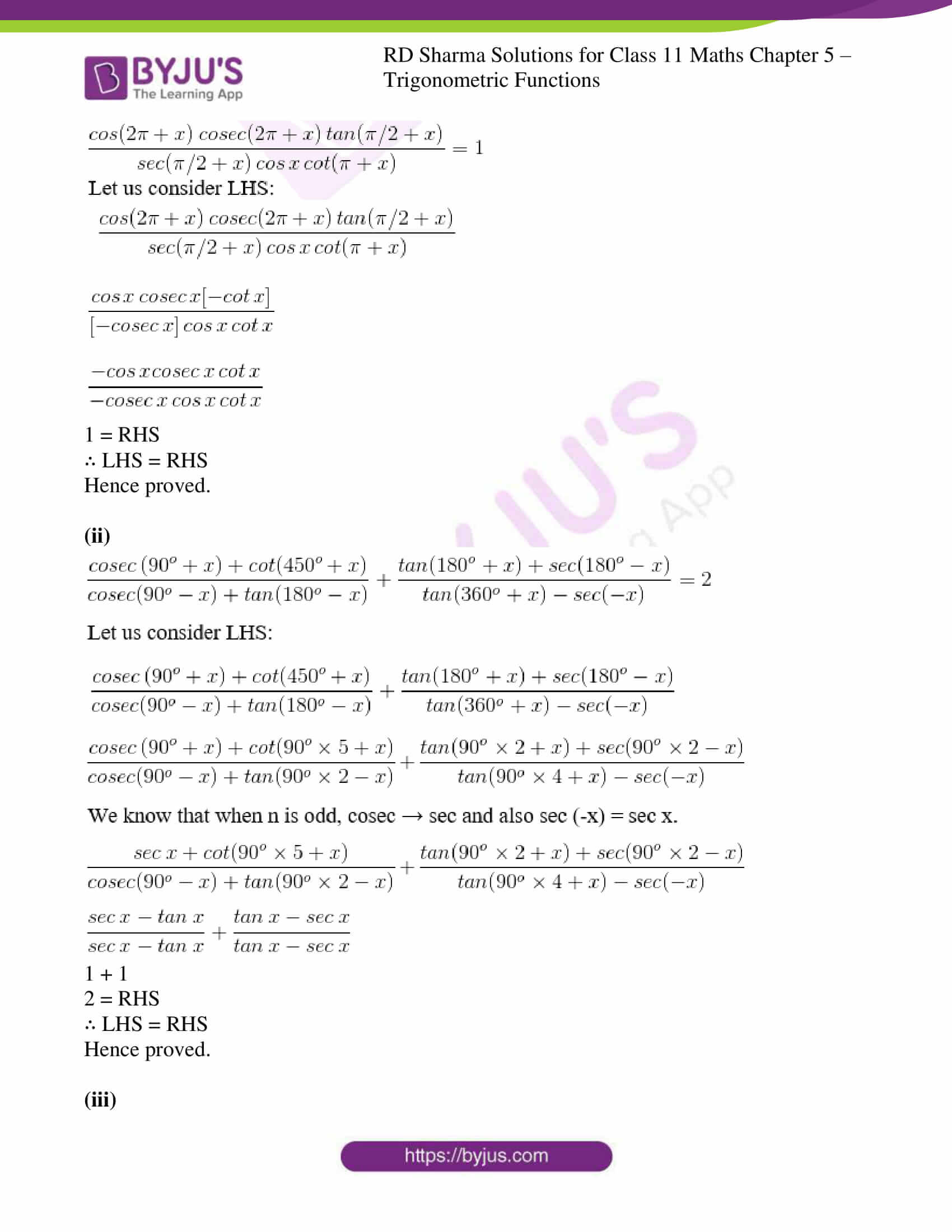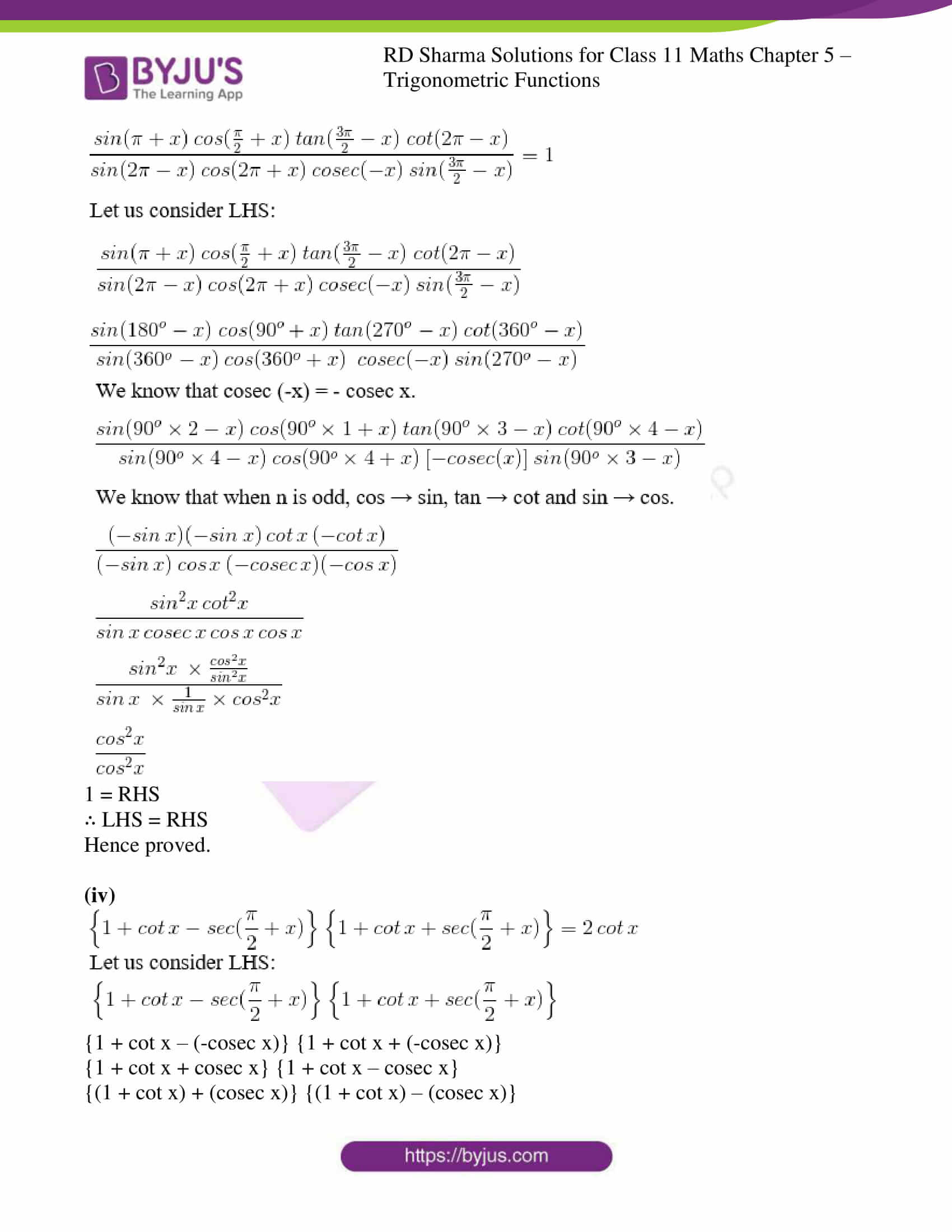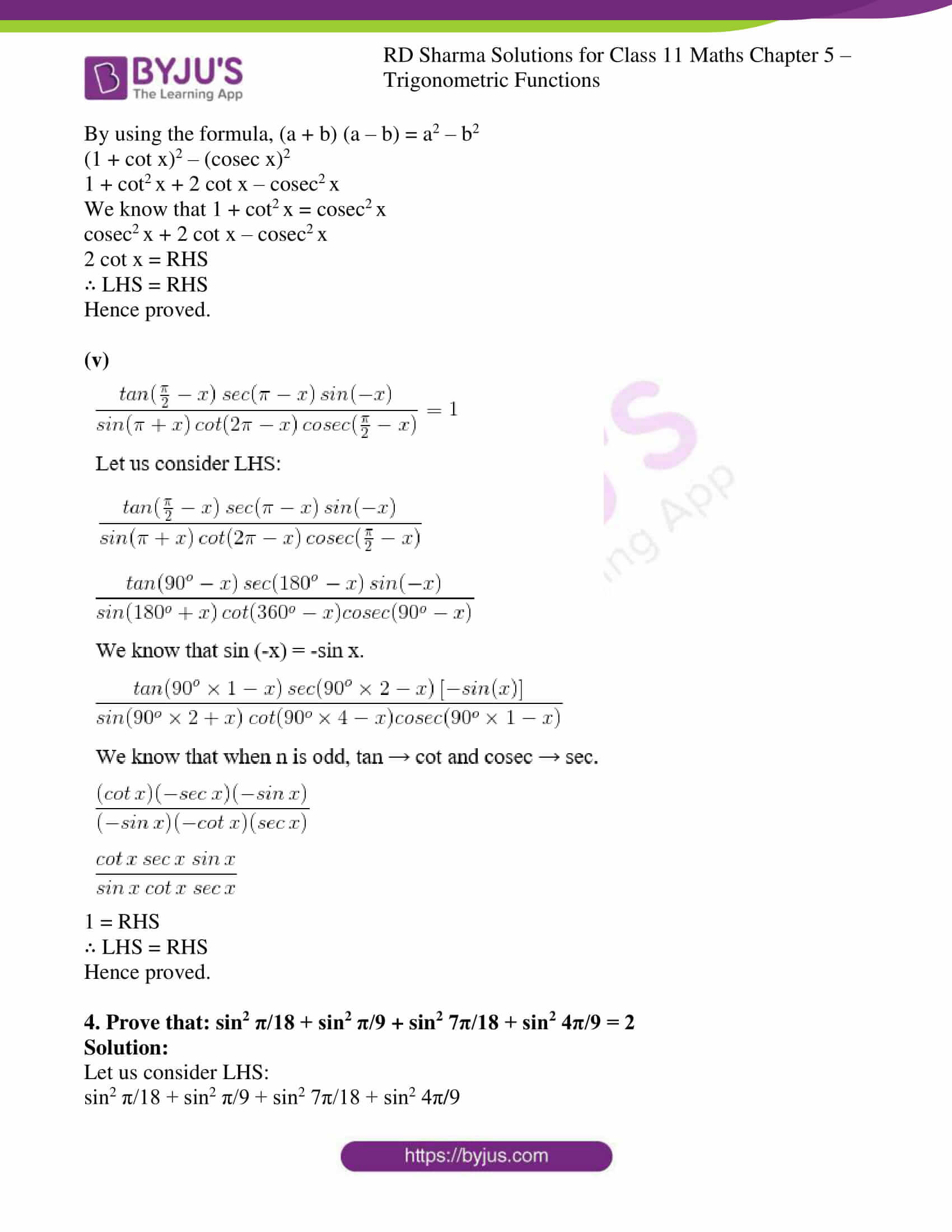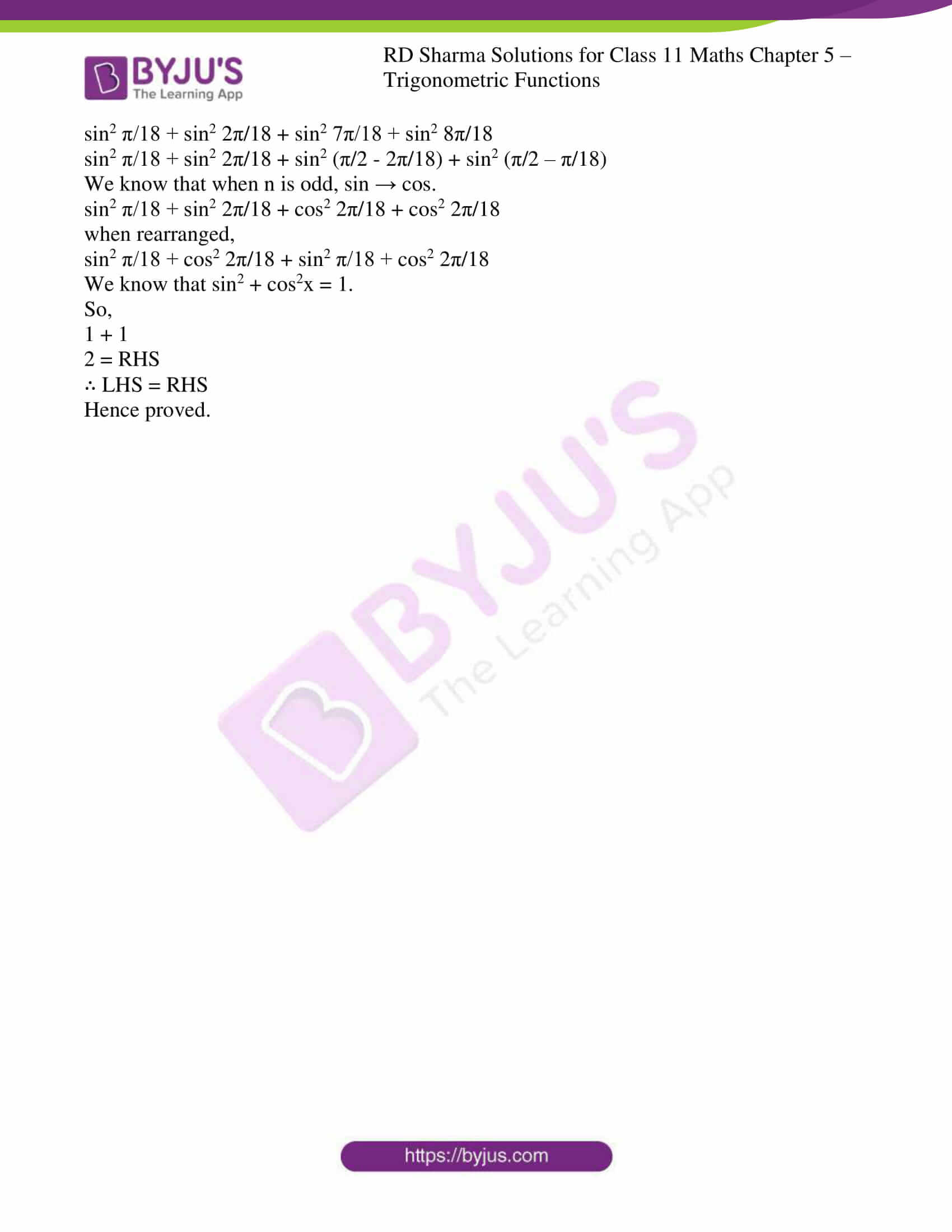Exercise 5.1

Exercise 5.2

### Access answers to RD Sharma Solutions for Class 11 Maths Exercise 5.3 Chapter 5 – Trigonometric Functions

1. Find the values of the following trigonometric ratios:

(i) sin 5π/3

(ii) sin 17π

(iii) tan 11π/6

(iv) cos (-25π/4)

(v) tan 7π/4

(vi) sin 17π/6

(vii) cos 19π/6

(viii) sin (-11π/6)

(ix) cosec (-20π/3)

(x) tan (-13π/4)

(xi) cos 19π/4

(xii) sin 41π/4

(xiii) cos 39π/4

(xiv) sin 151π/6

Solution:

(i) sin 5π/3

5π/3 = (5π/3 × 180)o

= 300o

= (90×3 + 30)o

Since, 300o lies in IV quadrant in which sine function is negative.

sin 5π/3 = sin (300)o

= sin (90×3 + 30)o

= – cos 30o

= – √3/2

(ii) sin 17π

Sin 17π = sin 3060o

= sin (90×34 + 0)o

Since, 3060o lies in the negative direction of x-axis i.e., on boundary line of II and III quadrants.

Sin 17π = sin (90×34 + 0)o

= – sin 0o

= 0

(iii) tan 11π/6

tan 11π/6 = (11/6 × 180)o

= 330o

Since, 330o lies in the IV quadrant in which tangent function is negative.

tan 11π/6 = tan (300)o

= tan (90×3 + 60)o

= – cot 60o

= – 1/√3

(iv) cos (-25π/4)

cos (-25π/4) = cos (-1125)o

= cos (1125)o

Since, 1125o lies in the I quadrant in which cosine function is positive.

cos (1125)o = cos (90×12 + 45)o

= cos 45o

= 1/√2

(v) tan 7π/4

tan 7π/4 = tan 315o

= tan (90×3 + 45)o

Since, 315o lies in the IV quadrant in which tangent function is negative.

tan 315o = tan (90×3 + 45)o

= – cot 45o

= -1

(vi) sin 17π/6

sin 17π/6 = sin 510o

= sin (90×5 + 60)o

Since, 510o lies in the II quadrant in which sine function is positive.

sin 510o = sin (90×5 + 60)o

= cos 60o

= 1/2

(vii) cos 19π/6

cos 19π/6 = cos 570o

= cos (90×6 + 30)o

Since, 570o lies in III quadrant in which cosine function is negative.

cos 570o = cos (90×6 + 30)o

= – cos 30o

= – √3/2

(viii) sin (-11π/6)

sin (-11π/6) = sin (-330o)

= – sin (90×3 + 60)o

Since, 330o lies in the IV quadrant in which the sine function is negative.

sin (-330o) = – sin (90×3 + 60)o

= – (-cos 60o)

= – (-1/2)

= 1/2

(ix) cosec (-20π/3)

cosec (-20π/3) = cosec (-1200)o

= – cosec (1200)o

= – cosec (90×13 + 30)o

Since, 1200o lies in the II quadrant in which cosec function is positive.

cosec (-1200)o = – cosec (90×13 + 30)o

= – sec 30o

= -2/√3

(x) tan (-13π/4)

tan (-13π/4) = tan (-585)o

= – tan (90×6 + 45)o

Since, 585o lies in the III quadrant in which the tangent function is positive.

tan (-585)o = – tan (90×6 + 45)o

= – tan 45o

= -1

(xi) cos 19π/4

cos 19π/4 = cos 855o

= cos (90×9 + 45)o

Since, 855o lies in the II quadrant in which the cosine function is negative.

cos 855o = cos (90×9 + 45)o

= – sin 45o

= – 1/√2

(xii) sin 41π/4

sin 41π/4 = sin 1845o

= sin (90×20 + 45)o

Since, 1845o lies in the I quadrant in which the sine function is positive.

sin 1845o = sin (90×20 + 45)o

= sin 45o

= 1/√2

(xiii) cos 39π/4

cos 39π/4 = cos 1755o

= cos (90×19 + 45)o

Since, 1755o lies in the IV quadrant in which the cosine function is positive.

cos 1755o = cos (90×19 + 45)o

= sin 45o

= 1/√2

(xiv) sin 151π/6

sin 151π/6 = sin 4530o

= sin (90×50 + 30)o

Since, 4530o lies in the III quadrant in which the sine function is negative.

sin 4530o = sin (90×50 + 30)o

= – sin 30o

= -1/2

2. prove that:
(i) tan 225o cot 405o + tan 765o cot 675o = 0

(ii) sin 8π/3 cos 23π/6 + cos 13π/3 sin 35π/6 = 1/2

(iii) cos 24o + cos 55o + cos 125o + cos 204o + cos 300o = 1/2

(iv) tan (-125o) cot (-405o) – tan (-765o) cot (675o) = 0

(v) cos 570o sin 510o + sin (-330o) cos (-390o) = 0

(vi) tan 11π/3 – 2 sin 4π/6 – 3/4 cosec2 π/4 + 4 cos2 17π/6 = (3 – 4√3)/2

(vii) 3 sin π/6 sec π/3 – 4 sin 5π/6 cot π/4 = 1

Solution:

(i) tan 225o cot 405o + tan 765o cot 675o = 0

Let us consider LHS:

tan 225° cot 405° + tan 765° cot 675°

tan (90° × 2 + 45°) cot (90° × 4 + 45°) + tan (90° × 8 + 45°) cot (90° × 7 + 45°)

We know that when n is odd, cot → tan.

tan 45° cot 45° + tan 45° [-tan 45°]

tan 45° cot 45° – tan 45° tan 45°

1 × 1 – 1 × 1

1 – 1

0 = RHS

∴ LHS = RHS

Hence proved.

(ii) sin 8π/3 cos 23π/6 + cos 13π/3 sin 35π/6 = 1/2

Let us consider LHS:

sin 8π/3 cos 23π/6 + cos 13π/3 sin 35π/6

sin 480° cos 690° + cos 780° sin 1050°

sin (90° × 5 + 30°) cos (90° × 7 + 60°) + cos (90° × 8 + 60°) sin (90° × 11 + 60°)

We know that when n is odd, sin → cos and cos → sin.

cos 30° sin 60° + cos 60° [-cos 60°]

√3/2 × √3/2 – 1/2 × 1/2

3/4 – 1/4

2/4

1/2

= RHS

∴ LHS = RHS

Hence proved.

(iii) cos 24o + cos 55o + cos 125o + cos 204o + cos 300o = 1/2

Let us consider LHS:

cos 24o + cos 55o + cos 125o + cos 204o + cos 300o

cos 24° + cos (90° × 1 – 35°) + cos (90° × 1 + 35°) + cos (90° × 2 + 24°) + cos (90° × 3 + 30°)

We know that when n is odd, cos → sin.

cos 24° + sin 35° – sin 35° – cos 24° + sin 30°

0 + 0 + 1/2

1/2

= RHS

∴ LHS = RHS

Hence proved.

(iv) tan (-125o) cot (-405o) – tan (-765o) cot (675o) = 0

Let us consider LHS:

tan (-125o) cot (-405o) – tan (-765o) cot (675o)

We know that tan (-x) = -tan (x) and cot (-x) = -cot (x).

[-tan (225°)] [-cot (405°)] – [-tan (765°)] cot (675°)

tan (225°) cot (405°) + tan (765°) cot (675°)

tan (90° × 2 + 45°) cot (90° × 4 + 45°) + tan (90° × 8 + 45°) cot (90° × 7 + 45°)

tan 45° cot 45° + tan 45° [-tan 45°]

1 × 1 + 1 × (-1)

1 – 1

0

= RHS

∴ LHS = RHS

Hence proved.

(v) cos 570o sin 510o + sin (-330o) cos (-390o) = 0

Let us consider LHS:

cos 570o sin 510o + sin (-330o) cos (-390o)

We know that sin (-x) = -sin (x) and cos (-x) = +cos (x).

cos 570o sin 510o + [-sin (330o)] cos (390o)

cos 570o sin 510o – sin (330o) cos (390o)

cos (90° × 6 + 30°) sin (90° × 5 + 60°) – sin (90° × 3 + 60°) cos (90° × 4 + 30°)

We know that cos is negative at 90° + θ i.e. in Q2 and when n is odd, sin → cos and cos → sin.

-cos 30° cos 60° – [-cos 60°] cos 30°

-cos 30° cos 60° + cos 60° cos 30°

0

= RHS

∴ LHS = RHS

Hence proved.

(vi) tan 11π/3 – 2 sin 4π/6 – 3/4 cosec2 π/4 + 4 cos2 17π/6 = (3 – 4√3)/2

Let us consider LHS:

tan 11π/3 – 2 sin 4π/6 – 3/4 cosec2 π/4 + 4 cos2 17π/6

tan (11 × 180o)/3 – 2 sin (4 × 180o)/6 – 3/4 cosec2 180o/4 + 4 cos2 (17 × 180o)/6

tan 660o – 2 sin 120o – 3/4 (cosec 45o)2 + 4 (cos 510o)2

tan (90° × 7 + 30°) – 2 sin (90° × 1 + 30°) – 3/4 [cosec 45°]2 + 4 [cos (90° × 5 + 60°)]2

We know that tan and cos is negative at 90° + θ i.e. in Q2 and when n is odd, tan → cot, sin → cos and cos → sin.

[-cot 30°] – 2 cos 30° – 3/4 [cosec 45°]2 + [-sin 60°]2

– cot 30° – 2 cos 30° – 3/4 [cosec 45°]2 + [sin 60°]2

-√3 – 2√3/2 – 3/4 (√2)2 + 4 (√3/2)2

-√3 – √3 – 6/4 + 12/4

(3 – 4√3)/2

= RHS

∴ LHS = RHS

Hence proved.

(vii) 3 sin π/6 sec π/3 – 4 sin 5π/6 cot π/4 = 1

Let us consider LHS:

3 sin π/6 sec π/3 – 4 sin 5π/6 cot π/4

3 sin 180o/6 sec 180o/3 – 4 sin 5(180o)/6 cot 180o/4

3 sin 30° sec 60° – 4 sin 150° cot 45°

3 sin 30° sec 60° – 4 sin (90° × 1 + 60°) cot 45°

We know that when n is odd, sin → cos.

3 sin 30° sec 60° – 4 cos 60° cot 45°

3 (1/2) (2) – 4 (1/2) (1)

3 – 2

1

= RHS

∴ LHS = RHS

Hence proved.

3. Prove that:

(i)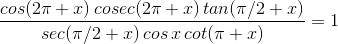(ii)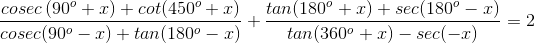(iii)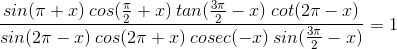(iv)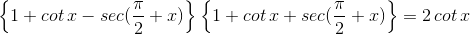(v)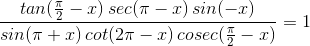Solution:

(i)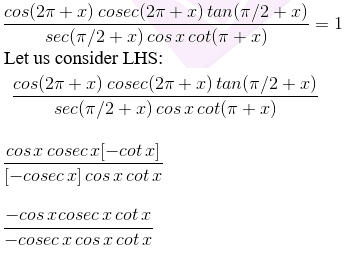1 = RHS

∴ LHS = RHS

Hence proved.

(ii)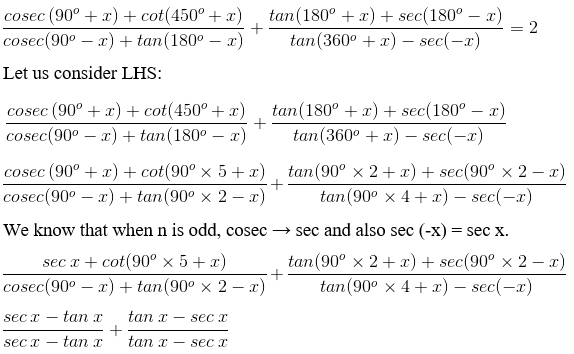1 + 1

2 = RHS

∴ LHS = RHS

Hence proved.

(iii)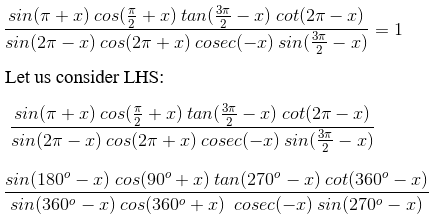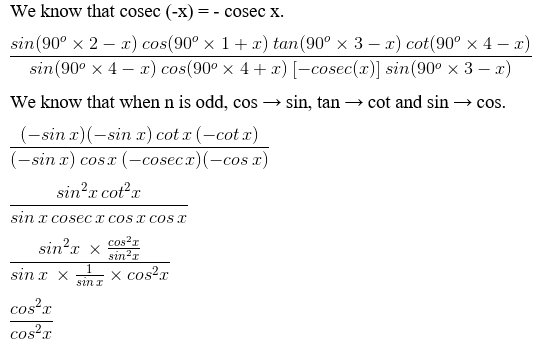1 = RHS

∴ LHS = RHS

Hence proved.

(iv)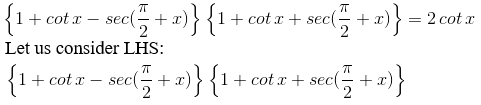{1 + cot x – (-cosec x)} {1 + cot x + (-cosec x)}

{1 + cot x + cosec x} {1 + cot x – cosec x}

{(1 + cot x) + (cosec x)} {(1 + cot x) – (cosec x)}

By using the formula, (a + b) (a – b) = a2 – b2

(1 + cot x)2 – (cosec x)2

1 + cot2 x + 2 cot x – cosec2 x

We know that 1 + cot2 x = cosec2 x

cosec2 x + 2 cot x – cosec2 x

2 cot x = RHS

∴ LHS = RHS

Hence proved.

(v)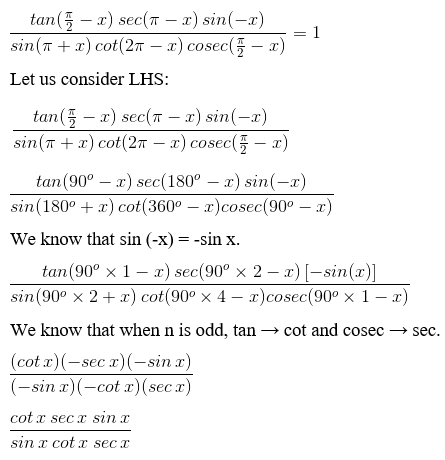1 = RHS

∴ LHS = RHS

Hence proved.

4. Prove that: sin2 π/18 + sin2 π/9 + sin2 7π/18 + sin2 4π/9 = 2

Solution:

Let us consider LHS:

sin2 π/18 + sin2 π/9 + sin2 7π/18 + sin2 4π/9

sin2 π/18 + sin2 2π/18 + sin2 7π/18 + sin2 8π/18

sin2 π/18 + sin2 2π/18 + sin2 (π/2 – 2π/18) + sin2 (π/2 – π/18)

We know that when n is odd, sin → cos.

sin2 π/18 + sin2 2π/18 + cos2 2π/18 + cos2 2π/18

when rearranged,

sin2 π/18 + cos2 2π/18 + sin2 π/18 + cos2 2π/18

We know that sin2 + cos2x = 1.

So,

1 + 1

2 = RHS

∴ LHS = RHS

Hence proved.